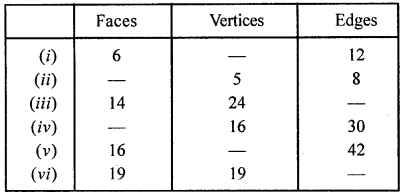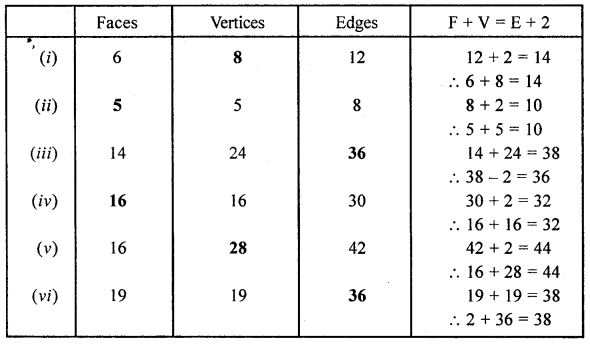## ML Aggarwal Class 8 Solutions for ICSE Maths Chapter 17 Visualising Solid Shapes Ex 17.2

Question 1.
Can a polyhedron have for its faces
(i) 3 triangles?
(ii) 4 triangles?
(iii) a square and four triangles?
Solution:
(i) No
(ii) Yes
(iii) Yes

Question 2.
Which are prisms among the following?Solution:
Prisms are only (i) and (iv).

Question 3.
Verify Euler’s formula for these solids:Solution:Question 4.
Can a polyhedron have 15 faces, 30 edges and 20 vertices?
Solution:
Can a polyhedron have 15 faces, 30 edges and 20 vertices.
∵ F + V = E + 2
⇒ 15 + 20 = 35 and 30 + 2 = 32
∵ 35 ≠ 32
∴ It has not.

Question 5.
If a polyhedron has 8 faces and 8 vertices, find the number of edges.
Solution:
A polyhedron has 8 faces and 8 vertices.
∴ Number of edges = F + V – 2 = 8 + 8 – 2 = 14

Question 6.
If a polyhedron has 7 faces and 10 vertices, find the number of edges.
Solution:
A polyhedron has 7 faces and 10 vertices.
∴ Number of edges = F + V – 2 = 7 + 10 – 2 = 15

Question 7.
Write the number of faces, vertices and edges in
(i) an octagonal prism
(ii) decagonal pyramid.
Solution:Question 8.
Using Euler’s formula, complete the following table:Solution: